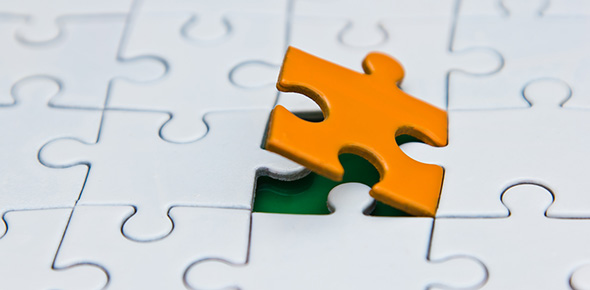# Chem Mastery Formula Mass Set 3

3 QuestionsSettings• 1.
What is the approximate molecular/formula mass of the following compound: Sn(CH3CO2)2
• A.

237

• B.

239

• C.

241

• D.

243

• E.

245

• F.

247

• 2.
What is the approximate molecular/formula mass of the following compound: Ca3(PO4)2
• A.

306

• B.

308

• C.

310

• D.

312

• E.

314

• 3.
What is the approximate molecular/formula mass of the following compound: MgSO4.7H2O
• A.

238

• B.

240

• C.

242

• D.

244

• E.

246